# Coefficient of Variation (%CV) Control Chart

Traditional variables control charts monitor two parameters or statistics - central tendency and variability. These are usually expressed using subgroup averages and subgroup ranges. One of the statistical assumptions regarding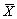and Range charts is that the subgroup mean is independent of the subgroup range.

There are some situations, however, where the mean and the variability are not independent. In these cases, another statistic, called the percent Coefficient of Variation (%CV), can be used to quantify the variation in the measurements.

### Percent Coefficient of Variation Plot Point

The %CV plot point is the subgroup sample standard deviation divided by the subgroup mean, multiplied by 100. In effect, %CV is the percentage of the mean represented by the standard deviation - a relative measure of variation.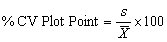Where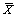is the subgroup average and s is the subgroup standard deviation.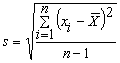Where i is the individual measurement and n is the subgroup size.

### Where Percent Coefficient of Variation Charts are Used

One example where %CV charts are used is in the textile manufacturing industry. The variation among tensile strength measurements from thin thread is significantly smaller than measurements taken from heavy thread. This is due to the inherent physical properties of fiber. Another use of the %CV statistic is to compare items that have different means, but share a common relative variation. Comparing multiple solutions with different chemical concentrations compounded in the same mixing line may be best analyzed using the %CV statistic.

### Specified Control Limits and Centerline

The centerline and control limits on the %CV chart are calculated based off the standard deviation chart. For specified limits the calculations are: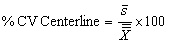Where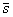is the specified centerline of the data displayed on the standard deviation chart and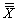is the specified mean as defined in the control limit record.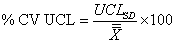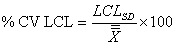Where UCLSD and LCLSD are the specified control limits from the standard deviation chart andis the process mean as defined in the control limit record.

### Calculated Control Limits and Centerline

The centerline and control limits on the %CV chart are calculated based off the standard deviation chart. For calculated limits the calculations are: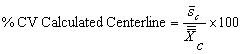Where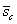is the calculated centerline of the data displayed on the SD chart and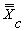is the calculated mean as defined in the control limit record.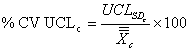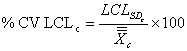Where UCLSDc and LCLSDc are the calculated control limits from the standard deviation chart andis the calculated centerline on thechart.

### Control Chart Display

Using ProFicient, any Range or Sigma control chart can be converted to a %CV chart. Simply go to the Chart Type dialog box (Chart>Configuration>Type menu item) and change Graph 2 to %CV. To display only the %CV chart, go to the Graph Setup dialog box and hide the top chart (theor IX chart).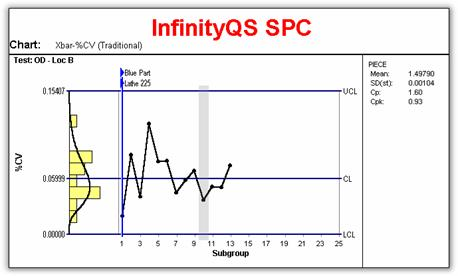%CV chart displayed without the corresponding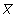Need help?
For further information contact GetInTouch@infinityqs.com
Toll Free: 1.800.772.7978

### Take the first step from quality to excellence

Take the Next Steps